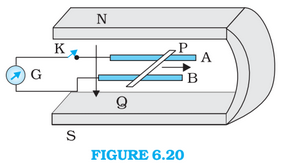# Q6.14 (e) Figure 6.20 shows a metal rod   resting on the smooth rails  and positioned between the poles of a  permanent magnet. The rails, the rod, and the magnetic field are in three mutual perpendicular directions. A galvanometer  connects the rails through a switch . Length of the , , resistance of the closed loop containing the . Assume the field to be uniform. Suppose K is open and the rod is moved with a speed of  in the direction shown. Give the polarity and magnitude of the induced emf.(e) How much power is required (by an external agent) to keep the rod moving at the same speed      when is closed? How much power is required when is open?Force on the rod

Speed of the rod

Power required to keep moving the rod at the same speed

Hence required power is 9mW.

When the key is open, no power is required to keep moving rod at the same speed.

Exams
Articles
Questions